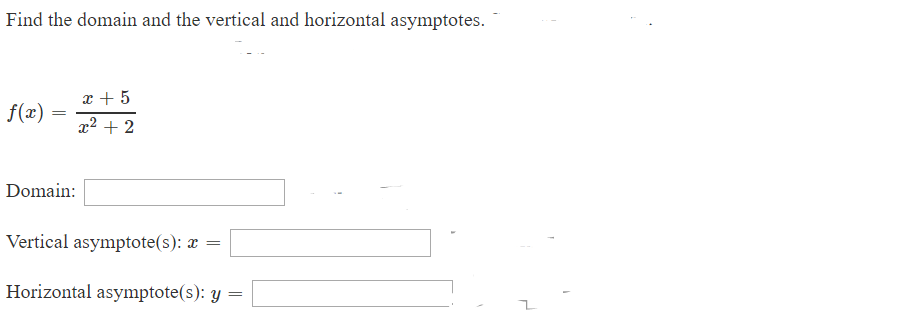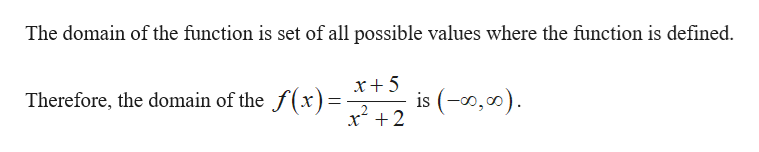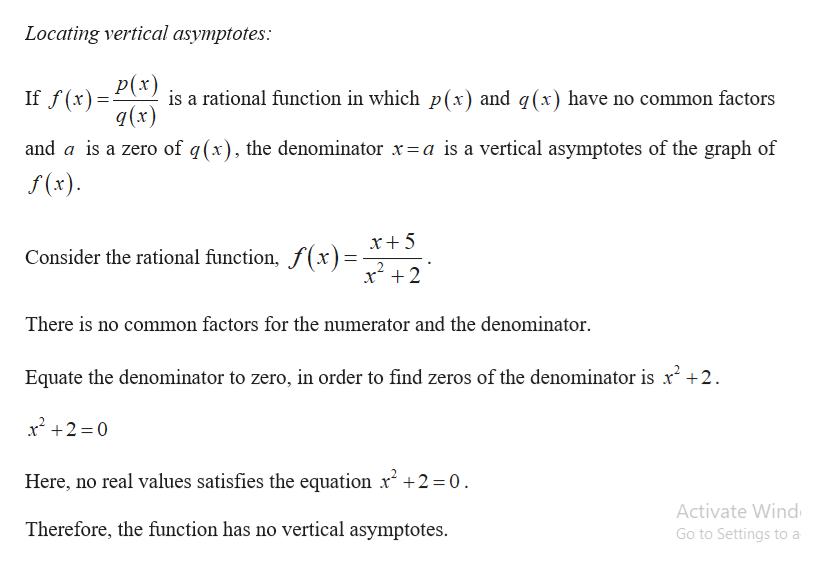# Find the domain and the vertical and horizontal asymptotes5f(x)22Domain:Vertical asymptote(s): xHorizontal asymptote(s): y

Question
1 viewshelp_outlineImage TranscriptioncloseFind the domain and the vertical and horizontal asymptotes 5 f(x) 22 Domain: Vertical asymptote(s): x Horizontal asymptote(s): y fullscreen
check_circle

Step 1

The function is given by,

Step 2

Find the domain of the function.help_outlineImage TranscriptioncloseThe domain of the function is set of all possible values where the function is defined x+5 is (,00) x2 Therefore, the domain of the f(x)= fullscreen
Step 3

Find the vertical ...help_outlineImage TranscriptioncloseLocating vertical asymptotes: P(x) is a rational function in which p(x) and q(x) have no common factors q(x) If f(x) and a is a zero of q(x), the denominator x=a is a vertical asymptotes of the graph of f(x) x+5 Consider the rational function, f(x)= x2 There is no common factors for the numerator and the denominator Equate the denominator to zero, in order to find zeros of the denominator is r2 +2 x22 0 Here, no real values satisfies the equation x2 +2 =0 Activate Wind Therefore, the function has no vertical asymptotes Go to Settings to a fullscreen

### Want to see the full answer?

See Solution

#### Want to see this answer and more?

Solutions are written by subject experts who are available 24/7. Questions are typically answered within 1 hour.*

See Solution
*Response times may vary by subject and question.
Tagged in

### Other